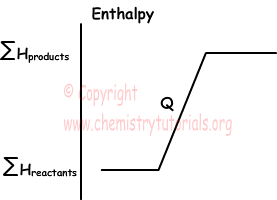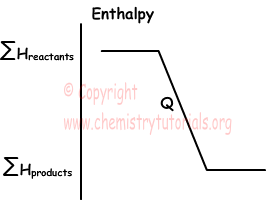EN | ES | DE | NL | RU

## Enthalpy and Thermochemical Reactions

Enthalpy and Thermochemical Reactions

Physical and chemical changes are done under constant pressure. Gained or lost heat in reactions under constant pressure is called enthalpy change. Enthalpy is the total kinetic and potential energy of particles of matter. It is denoted by letter "H". Enthalpy of matters can not be measured, however, enthalpy change can be measured. We can find change in enthalpy as given below;

Reactants → Products

If HR is the enthalpy of reactants and HP is the enthalpy of products, change in enthalpy becomes,

∆H=HP-HR

• In exothermic reactions, HR is larger than HP, so enthalpy change becomes negative

HP<HR so; ∆H<0

• Since endothermic reactions absorb heat, HP>HR and enthalpy change becomes positive.

HP>HR so; ∆H>0

• Enthalpy change depends on temperature and pressure. Thus, you should compare enthalpy changes of reactions under same temperature and pressure.
• Enthalpy change under 1 atm pressure and 25 0C temperature is called standard enthalpy change.

In endothermic reactions, enthalpy of system increases. For example, enthalpy of water is larger than enthalpy of ice at same temperature.Graph given below shows enthalpy of endothermic reactions;In exothermic reactions;Thermochemical Reactions

Reactions showing both changes of matters and energy are called thermochemical reactions. Examples of thermochemical reactions;

• Exothermic reaction;

C(s) +O2(g) → CO2(g) ; ∆H=-94 kcal

This reaction tells us that, 1 mol C(s) reacts with 1 mol O2(s) and produce 1 mol CO2, and 94 kcal heat released.Reaction becomes;

C(s) +O2(g) → CO2(g) + 94 kcal

• Endothermic reaction;

2H2O(g) → 2H2(g) + O2(g) ; ∆H=116 kcal

This reaction explains us, 2 mol H2O absorbs heat and decompose into 2 mol H2 and O2.

2H2O(g)  + 116 kcal → 2H2(g) + O2(g)

Properties of Thermochemical Reactions

• Coefficients in front of each element shows number of moles of matters and given ∆H value shows heat released or absorbed by reaction balanced with these numbers.

Example: Find heat released from reaction in which 2 mol CH4 and 2 mol Cl2 react to form CCl4 and HCl.

CH4(g) 4Cl2(g) → CCl4(g) + 4HCl(g) + 104 kcal

Solution:

Reaction given above is balanced for 1 mol CH4, we should find limiting matter first.

1 mol CH4 react with 4 mol CCl4

? mol CH4 react with 2 mol CCl4

¯¯¯¯¯¯¯¯¯¯¯¯¯¯¯¯¯¯¯¯¯¯¯¯

?=0,5 mol CH4

2-0,5=1,5 mol CH4 is not used in this reaction since CCl2 is limiting matter.

Heat releases from reaction is calculating by considering limiting matter;

4 mol Cl2 release 104 kcal heat

2 mol Cl2 release ? kcal heat

¯¯¯¯¯¯¯¯¯¯¯¯¯¯¯¯¯¯¯¯¯¯¯¯

?=52 kcal heat is released from the reaction of 2 mol Cl2.

• If you multiply reaction with number "n" than you must multiply ∆H value also with "n".

Example:

CO(g) + 1/2O2(g) → CO2(g) ; ∆H=-68 kcal

When we multiply reaction with 2;

2CO(g) + O2(g) → 2CO2(g) ; ∆H=2(-68)=-136 kcal

• If direction of thermochemical reaction is changed, then sign of ∆H is also changed.

Example:

2H2O(g) → 2H2(g) + O2(g)   ∆H=116 kcal

2H2(g) + O2(g) → 2H2O(g)   ∆H=-116 kcal

As you can see from the example, when we change direction of reaction, sign of enthalpy change also changes.

• Since ∆H depends on states of matters, you must write states of matters in thermochemical reactions.

H2(g) + 1/2O2(g) → H2O(g)   ∆H=-58 kcal

H2(g) + 1/2O2(g) → H2O(l)    ∆H=-68 kcal

As you can see from the examples, enthalpy of water at liquid state is lower than enthalpy of water at gas state.

Thermochemistry Exams and  Problem Solutions

The Original Author: